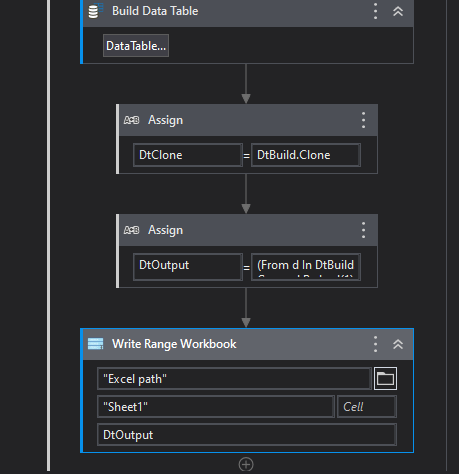# How to find 2 column's duplicate records in excel?

Hi everyone!

I want to find duplicate records. I have to look 2 cloumns in excel.
Column B and Column D must match each other. How can I do it?

Can you share the Output here

You can try with LINQ

``````(From d In DtBuild.AsEnumerable
Group d By k=d(1).toString.Trim, k2=d(3).ToString.Trim Into grp = Group
Let ra = New Object(){grp.First()(0),k,grp.First()(2),k2}
``````

Regards
Gokul

Hii Gokul!

The result should be like this.

Can you explain this query more?

@ozgecatak
2.Use For each data table activity to loop the rows
2.1. Use if condition

``````CurrentRow(1).Tostring.trim=Currentrow(2).Tostring.trim
``````

2.2. If Both values is the same, the process will go to then sequence, otherwise else sequence.

1 Like

Regards
Gokul

1 Like

Check out the Skeleton XAML

GroupByLinq.xaml (8.4 KB)Regards
Gokul

Assign Activity
dtResult | DataType: DataTable =

``````(From d In dtOrigin.AsEnumerable
Group d By k1=d(1).toString.Trim, k2=d(2).ToString.Trim Into grp = Group
Where grp.Count > 1
Where k1.Equals(k2)
Select g = grp).SelectMany(Function (x) x).CopyToDataTable
``````

we group the rows
when group member count > 1
When

which we check with the key k1= k2

then we return the group members

[HowTo] LINQ (VB.Net) Learning Catalogue - Help / Something Else - UiPath Community Forum

1 Like

Checkout this expression

``````(From p in dt.Select() where( From q in dt.Select() where q("Column name1").Equals(p("Column Name 1")) and q("Column Name2").Equals(p("Column Name 2")) Select q).ToArray.Count>1 Select p).ToArray.CopyToDataTable()
``````

From p = p is an actual dt from that p.select where( From q “q is a clone dt”) .select from that
q(“Col1”).Equals(q(“Col1”) and q(“Col2”).Equals(q(“Col2”) select those values from q . ToArray.Count>1 Select those values from p datatable ).ToArray. Copy those values to datatable

Regards
Sudharsan

This topic was automatically closed 3 days after the last reply. New replies are no longer allowed.